# The Hexagonal Prism

The hexagonal prism is a 3D uniform polyhedron bounded by 8 polygons (2 hexagons and 6 squares), 18 edges, and 12 vertices. It is the extrusion of the hexagon into 3D.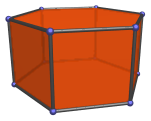Attaching a triangular cupola to a hexagonal prism produces the elongated triangular cupola (J18), a Johnson solid. Attaching a second triangular cupola produces either the elongated triangular orthobicupola (J35) or the elongated triangular gyrobicupola (J36), depending on whether the second cupola is aligned or rotated with respect to the first, respectively.

The hexagonal prism can be augmented by up to 3 square pyramids to produce a series of convex augmented prisms, which are Johnson solids:

## Projections

In order to be able to identify the hexagonal prism in various projections of 4D objects, it is useful to know how it appears from various viewpoints. The following are some of the commonly-encountered views:

Projection Envelope Description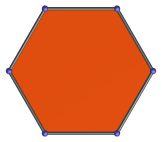Regular hexagon

Hexagon-centered parallel projection.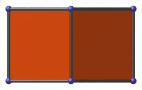Rectangle

Parallel projection centered on a vertical edge.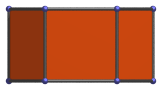Rectangle

Square-centered parallel projection.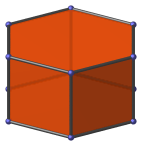Hexagon

Vertex-centered parallel projection.

## Coordinates

The Cartesian coordinates of the hexagonal prism, centered on the origin and having edge length 2, are:

• (±√3, ±1, ±1)
• (0, ±2, ±1)

## Occurrences

The hexagonal prism occurs as cells in the following 4D uniform polytopes:

It also occurs in a number of CRF polychora, including (but not limited to):

Last updated 17 Jun 2019.